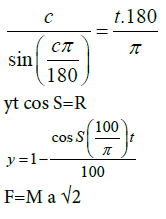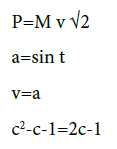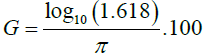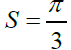Astro-Theology, Cusacks Universe | OMICS International
Journal of Physical Mathematics

# Astro-Theology, Cusacks Universe

Cusack P*

*Corresponding Author:
Cusack P
Independent Researcher
Tel:
(506) 214-3313
E-mail: [email protected]

Received Date: February 08, 2016; Accepted Date: May 24, 2016; Published Date: June 05, 2016

Citation: Cusack P (2016) Astro-Theology, Cusacks Universe. J Phys Math 7: 174. doi: 10.4172/2090-0902.1000174

Copyright: ©2016 Cusack P. This is an open-access article distributed under the terms of the Creative Commons Attribution License, which permits unrestricted use, distribution, and reproduction in any medium, provided the original author and source are credited.

Visit for more related articles at Journal of Physical Mathematics

#### Abstract

This paper presents a new revolutionary theory on how the universe came to be and is sustained. It shows, through the use of Mathematical Physics, how the so-called “Superforce is” determined. The Superforce is that single force that overrides all other forces of Physics, namely, Gravity, Electromagnetic, and Nuclear Forces. The theory presented here is in harmony with other field that touch on it, including Quantum Mechanic, Chemistry, Physics, Theology, Sacred Scripture, and Philosophy. Newtonian Mechanics is useful here. There is absolute time and space contrary to Einsteinian Mechanics. Our universe is just one wavelength of a spectrum. Shown is the irreducible gravitational constant and how the 11 dimension universe is built up from there. The paper provides a solution to these 11 simultaneous equations. This paper is a brief summary of the blog Astrotheology-Cusack’s Universe and the series of books by the same title. That blog is over 4300 pages posts long, and thus difficult to summarize in less than 20 pages. However, the attempt is made here. The reader is referred to those sources to glean the detail. This paper bring physics into harmony with Quantum Mechanics and also with the account of Genesis account version of Creation, which the Egyptians knew and the Hebrews learned The ancients knew much more about our universe than we previously thought.

#### Keywords

Barriers to cessation; Reasons for quitting; Cigarette; Drinking; Concurrent

#### Introduction

Genesis, creationism, and the energy time parabola and the golden mean

Genesis records the Creation "Myth" at the very outset of the Bible. Where did this “myth” come from? I was surprized to learn that the Egyptians knew of the quadratic equation. This, of course, is the equation that is used to solve a polynomial. While studying the layout of the great Egyptian Pyramids, I could see that were laid out from above alone a parabola (Figure 1). This parabola happens to be the Golden Mean parabola. The Golden Mean is 1.618 and its conjugate 0.618 are important in the construction of the Pyramids. The ratio of the height to the base is 1.618. So why is the Golden Mean important .

The answer to this is that it is a number somewhat like π or base e. It is an infinite number that solves the equation x=1/(x-1). It is where the fraction meets the multiple. If we multiple this equation out, we get the famous polynomial, x2-x-1. In fact, if we let x-t, we have the first equation of 11 that is a set that solves the physical universe. So, t2-t-1=0 is our first equation. Refer to Figure 2 for the plot of the Golden Mean equation.

The Hebrew spent approximately 400 years in Egypt when joseph, the patriarch, was sold into slavery by his brothers. After the Pharos learned Joseph could interpret dreams, the Pharos put Joseph second in command on the Egyptian Empire. Now, Moses was educated in the royal household of the Pharos. If you recall, Moses was put in a basket and floated down the stream, until he was picked up by the Queen and raided in the Kingly household. Moses is the one who recorded the Book of Genesis. So the Genesis Creation “Myth” was based on the Egyptian mathematical understanding of the universe and recorded in the dimensions of the Pyramids in 2200 BC (Figures 1 and 2).

The Energy-time parabola results when the Energy, time plane rotates through a conic section (Figure 3). The Conic Section results from the Energy spiral. In our universe, y=y’ or the derivative equals the function. The function which has its derivative equal to the function itself is the exponential function y=ex. Total Energy is a negative exponent of this exponential function. When the angle of rotation is just right, the Energy-time parabola is satisfied and the material universe comes (Figure 3).

So the Energy–time plane is the essence of the material universe. It is formed by 11 equations that describe 11 facets or dimensions of our universe, discussed below. This vector Space reduces to components Energy and time. The rotation of the Energy –time plane is critical at 60°. Why is this so? We need to turn to Soil Mechanics for the answer.

Mohrs circle, eigenvector and eigenvalue

In Soil Mechanics, as well as Mechanics of Materials, Mohr’s Circle is an easy way to illustrate the failure place of a soil mass. It is a plot of the shear stress versus the normal stress. The line tangent to the half circle gives the angle of the failure plane beyond which the soil is stable. Why look at Soil Mechanics when dealing with the universe.

The answer to that question is that the universe is made up of soli particles, which is called “dust” in Genesis. “For Man was made of dust, “the Bible words. Actually, the universe is made of small particles or dust, and man is made from the universe, so man indirectly is made from dust.

When the shear stress to normal tress sat a 60° failure plane, the universal material fails and begins to flow. It becomes a fluid, or the Genesis states, “the waters above and the waters below.”

The 60° is inherent in the universal material. It is actually kaolinite, which is a common type of clay. This is discussed below (Figures 4 and 5).

The ellipsoid, Kalonite universe

Below are Figures of the Kaolinite particle which as mentioned, make up the universal material. When the Energy input into the universal material is 0.202, the Reynold’s number for the material is overcome and the material becomes a fluid (Figure 6).

Figure 7 shows a Kaolinite particle surrounded by a fluid – the universal fluid. Its dimension is 0.1420 whose conjugate is 0.858. This number, 0.858 is an important Energy value since is the energy input into the universal ellipsoid.

What is important form the Kaolinite is that the rupture plane is 60°. We will show how this manifests itself in the creation of the material universe .

Rupture occurs when the failure plane is at 60°. This means, from the geometry, that there is a 30-60-90 triangle which, of course, has a hypotenuse of 2,a base of 1 and and a height of √3. When the base is time=1, the height is √3. The √3 is the eigenvector for the universal system, Its eigen value is 3 which happens to be the speed of light or, the speed of Electromagnetic radiation in general (Figure 8).

The cross product of Energy and time at 60° is space, s=4/3 or 1.334. Refer to Figure 9.

This is important when determining the value for the irreducible gravity G. The volume of the trapezoid is (1) * (1) * (1.334) * 1.2=0.66=G. Refer to Figure 10.

When the value of the Gravitational Constant is 6.67, and when it is divide by the space, 4/3, the Energy level at E=2 (recall 30-60-890 triangle hypotenuse), the universal material overcomes its resistance to flow and mass precipices.

Hookes law and resitance to mass formation

The universal material has a Modulus of Elasticity or Young’s Modulus of π-base e=3.141-2.718=0.4233.

I dub this value “cuz”. Mass is formed when Energy is compressed. It is compressed by the Superforce pushing down on the “exterior” of the universal ellipsoid. Refer to Figure 11. Since the Total Energy is given by et, and the Kinetic Energy is given by Ln t, when t=π, the Resistance to Mass formation overcome and the mass precipices. Refer to Figure 12.

Superforce and four forces

So far, we have Energy, time, space, Gravity, Resistance to Mass, Force, speed of light.

There is Period (T=1/t), Momentum (P=Mv), frequency of human perception=31.8 Hz=1/π, (freq=1/t), and N dimensions ((1+t) N)

The 11 simultaneous equations are:s=1-a

M+m=10√s

πr+πr2=Ym=tan SAnd their solutions are:

c=3.0

y=.840772

S=1.0472

R=.420578

t=1.00046

F=2.67407

M=2.24642

a=.841718

v=.841718

P=2.67407

s=.158282

m=1.73205

Y=14.8662

r=1.73205

G=6.65199

The universe is the shape of an ellipsoid, or a flattened cigar. The reason is that the superforce compressing the ellipsoid fails when the tan θ=7.125°. This makes a 1 x 8 ellipse (or 3 x 24). Since the volume is 19905, the ellipsoid must be 22 long (or 66) (Figures 13 and 14).

Whereas the stress cube shows that when Force=2.667 (or 8/3), the Gravitational Constant must be 6.67. (This includes the value of the weak nuclear forces) Refer to Figure 15.

The other two forces in the universe are the strong nuclear which results from the Superforce/e-=2.667/1.602=1/6. This is the ratio of the Area of a circle/Circumference=2πR/πR2=1/6

2R=6

R=3

This is the top dimension, 1 LY.

Clairnaut equation and gravity

The only force there need be is Gravity (Figure 16). As the Sand accumulates, it pushes down on the universal ellipsoid. The force causes Mass to form, since when you squeeze Energy, you get Mass . The force is of course, is sin θ. This is equal to Newton's Formula F=G M1M2R2

We know from above that

G/Ln (1.618)/π

So F=(6.65)(4.486)(1)/12=sin θ

θ=0.173=-√3=√c=(eigen vector)

This eigen vector leads to space since √[12 x 12 x 12]=√3

At 60°, s=|E||t|sin 60=0.866=√2

From the Clairnaut, we know,

D2E/dt2 – E=0

G-E=0

So, π/1`.618)- e/(1.618)=(π-e)/1.618=2616

t-E)/1.618)=2616(1.618)=π-e

G-E=t/Ln t - E=0

t/Lnt=E

t=E

When you have time and Energy equal, you will necessarily have, G, M, θ, F, a, P, v, s, and thus N So Gravity is all you need to have a universe., Gravity is Energy and we have shown is is irreducible with y=y'

The Universe had no choice but to exist. Why? Because the derivative of y=et itself. The rate of change of Energy with respect to time is Energy. It is robust. So, by Euler's Identity,

et=cos t+i sin t, the left hand side had to exist, so the right hand side had to exist. Therefore, Force and it rate of change, Momentum had to exist. Mass had to exist because is Energy Compressed by that force. There is no question that our universe had to exist. Genesis states that The Spirit of God, (Energy) hovered over the waters (fluids)=mass. Interesting that E=Mc2. Because the next thing Genesis that came to be was light. God said, Let there be light. So, we find the Theologian and Physicists at the top of the Mountain, together (The Philosopher is still trying to determine which way is up the mountain) .

#### Irreducible gravity

The reason Gravity is so weak is that Mass is so small (4.486) and Volume is so large (19905).

F=GM1M2/r2

=6.67(4.486)

=2.9979

=c Eigen value

Electromagnetic Spectrum Speed is slowed by the density (127) of

the Mass spread throughout the universal ellipsoid.

sin2 θ=F=sin2 2 (2.9979)

θ=√3=E eigenvector

So the eigen vector is √3 because the failure plane is 60° by the geometry of the compressed matter spheres. The question becomes why must Energy have Rm=cuz=π-e? The answer to that s that T.E=e-t and K.E=Ln π . π is the node of the Harmonic Beat. This is expressed by the Clairnaut Equation, d2E/dt2-E=0 where G=d2E/dt2 The Harmonic Beat at time=π causes the Gravity in the Clairnaut Equation to come into existence.

The other way to ask why is Gravity so weak is why are the eigen values and eigen vectors the values that they are? Why is the universe the size it is? And why is the force=sin θ so strong. These characteristics are inherent in the universe. Since these values are what they are, the universe necessarily exists.

Vector space

All 11 equations can be plotted in a Vector Space. Refer to Figure 17. The base of these of all these vectors is time and Energy, which brings us back to the rotating plane mentioned at the beginning.

Forces, momentum, and function–derivative

So growth and decay are balanced for maximum output with minimum expenditure by the Multiplier Effect in Economics. That is, for every dollar earned, 1/7 or 15% is saved. So \$1 is circulated 7 times before it all runs out (Figure 18).

It is the same in Physics. The T.E. is given by y=e-x and K.E. is given by ln x . The difference between them is the Mass Resistance overcome=π-e=0.4233.

Continuing, e*(1-0.14%)=e-0.86=e-sin 1 1/-sin 60°=1/-sin 1=2/√3=0.86

1/-0.86=(1-0.86) 1/x=(1-x)

Or The golden Mean Equation:

x=1/(x-1)

This is the solution to the universe (Figures 19 and 20).

The Beat, Inside and Outside the Universe

The harmonic beat is given in Figure 21.

#### Summary

So, we have dust that has gravitational attraction toward itself. As the dust accumulates, and tends more so, the pressure builds up in a cylinder. A wave is vibrating between the out universe and the inner universe. Actually, the inner universe comes into existence when the dust particles begin to flow like liquid. As the irreducible gravity results from the relative rotation of the Energy vector and time vector, and the resultant space (cross product), the universe in 11 dimensions is formed. There is a system of 11 equations and 11 unknowns. Now the Energy and time vector rotate because of a vibration of a harmonic string, resulting in the Clairnaut Equation which is G and E. It is a harmonic oscillation. the result is a dampened cosine curve inside the inner universe. When the resistance to Mass formation overcome in the failure plane of the Mohr's circle, the dust like particles flow. this flow results in mass that is cooling. The force on the outside of the inner universe is sin θ. The Total Energy is e-t. And that, my friends, is how the universe came to be. Because the derivative is equal to the function and spirals in on itself, there was no choice but that it had to exist. This exponential derivative is related to the sin and cosine functions and the imaginary number by Euler’s Identity. Time, one of the 11 equations, is governed by the Energy Parabola. The human mind is tuned to the universal frequency, 1/π. The wave is travelling at 10-23 seconds which is why the quantum particles disappear at this duration. the ones that don't are matter. Since Energy is a vector, it has a purpose or a direction. This is why quantum particles seem to follow the anticipated vital statistics. And that is CUSACK'S MODEL OF THE UNIVERSE! This is the mathematical way of saying the same thing recorded in Genesis in the time of Moses [6-10].

My interest in Mathematical Physics began with the problem of the termination of two infinite numbers, π and e. The solution is where the circumference of a circle equals the Area subtended by the circle. The both rely on π. Since the universe is a physical reality, π cannot logically be infinite. It terminates, as the Babylonians knew, at 6 and 1/6. This is shown below. The conclusion is that the Babylonians knew more Physics than we knew today, henceforth. This Tablet’s interpreted shows how much Mathematical Physics the Ancients knew. It is amazing that they knew so much. I don’t think we had any idea (Figure 22).

The Area of a circle is the derivate of the circumference of it. The rate of change of Area is equal to the circumference at R-=2. If we set circumference=2.9989, the speed of light according to the Babylonians,

Circ=2π R=2πR=2.9989 R=1.4995 * π=4.71=2+e=dM/ dt+e==1/2123

Or Feb 12 at 3 AM.

Since the universe is a physical reality, and it is dependent upon π, π must terminate at where the circumference equals the area of a circle. The Area and the circumference are both dependent upon π. 6 decimals are where π terminates. Engineers never need more than 6 significant digits.

I can read this tablet from Samaria YBC 289. It is a Babylonian Tablet that records all the Physics they knew.

30=03=c=speed of light

01 15=115=1.15=√3* 2/3=1.15=1/sin 60°, 1/F

1/51=196=∞

51/4.482=1138 1/1138

Permeability 885/51=1.73=√ 3=Eigenvector=t

0.885/4.482=01/51

42=cuz=-e

25 &35 are read backwards giving 5352. sin-1(0.5252)=32.35°. This

is 1/√π

32 in the cubed root ta

bles of Samaria=3.1744~π

freq0.333=31.80.333=3,16~π

And

0.5616=1/0.1781∗2π=0.1119=c2

c=2.9893 speed of light.

1 & 24 are read 421-2.718=1/254=1/Period T

421-271=0.666=G and therefore the Mass Gap

4.486/0.42/√2=0.868=sin 60°4.486/cuz/√2=0.1336=s

s=|E||t|sin 60°=60°

4.486/0.4233/√3=16=R

E, t, s, c G, M, T, freq, cuz,, e, M, F,t, R, θ, Eigenvector, Eigenvalue=c

This is what the Babylonians knew in 2000 BC E, or before. They knew all this Physics, which henceforth was not known till this day.

So how did the Babylonians know all this? They knew a lot more than just the √2 as suggested by Figure 23.

CU-SPACE rotated 45°, in profile, is the square with the double diagonals. They would be the vectors here. The area of the cones is 2∗π/2=π. Measuring the tablet will indicate the degree of accuracy of the Babylonian π. I think it may be to 6 decimals

Note the following:

π=3.14159=God

3*1/3*1*4*1/4*1*5*1*9/5 π to six decimals 3.14159=3

π/3=60°=Sexigesimal System

2π/3=0.666π

π evil, evil evil god

2*7/2*7*1/7*1*1/1*8*1/8*8/2*2*8

7/2*1*8*2/8*8/2

=4488

=Mass M=4.486

4.171=(2+e)

Ln (2.388)/Ln e=3.173/1=∗32~π

e/π=0.8653=sin 60°

3*1*4**1 *5*9=54

54/9=6 decimals.

Evil=6=54/9

5=, Holy Spirit, 4=me, 9=Holy Spirit

Figure 25: Soil Mechanics calculations using Mohr’s Circle for Shear and Vertical Stresses.

(5*4)/9=20/9=0.222=1/45

1/(4+5)=1/9==0.111=1/c2

I think this is a method to terminate an infinite number. It is where the circumference=Area of a circle.A’=circ=πR2=2πR R=2

A’=C=4π

Circ=2πR=3 (Termination of π)

R=4775

R (in reverse)=5774=1/√3=5774=tan 60°

3761 BCE -1 (No year zero) + 5774=2012

Christian Calendar is off by 4 years. (Christ born in 4 BC E, December 25)

A’=π(62)=36π=11.3097=1/0.884=Permeability

113.097/511=0.2213

Sin 0.2213=0.1278=ρ=Universal Density,

Hanging Gardens of Babylon (Nineveh): On another matter, the Ancient Hanging gardens of Babylon, were likely built in Nineveh, because Jonah was to preach there. Jesus said that “There is something more than Jonah here.” The Gardens would have been 81 feet high, since 1/81=0.12345679 related to the Mass of the universe. Or 24 m=7.87 feet 1/787=0.127=ρ. There would have been 7 levels 0-7. The other physical constants listed above would have been built into the structure symbolically. Here are possible calculations Babylonian Engineers would have made (Figures 24 and 25).

#### References

Select your language of interest to view the total content in your interested language

### Article Usage

• Total views: 9846
• [From(publication date):
June-2016 - Feb 29, 2020]
• Breakdown by view type
• HTML page views : 9644Can't read the image? click here to refresh